# Introduction to phylogenetic path analysis with phylopath

## Some starting notes

If you are unfamiliar with phylogenetic comparative methods in general, I’d advise you learn more simple methods first. If you are comfortable with phylogenetic methods, but not with phylogenetic path analysis (PPA) in particular, you should have a look at the example below together with the wonderful book chapter by Gonzalez-Voyer and Von Hardenberg.

### Starting up

This package follows the general approach to phylogenetic methods as used by the package phylolm (this package is used for model fitting “under the hood”). This means in particular that you line up your phylogenetic tree and data by assigning the tip labels as the row names of your data.frame. (Please note that this is the same for ape, but different from the approach of caper::pgls where you create a comparative data object.)

Your phylogenetic tree needs to be a phylo object, and many tree files can be read into R using the ape package (which is installed automatically with phylopath). For example:

library(ape)
my_tree <- read.tree('my_tree.tre') # For Newick format trees
my_tree <- read.nexus('my_tree.nex') # For NEXUS format trees

If you have a column in your data that contain the species names, you have to set those names are rownames, e.g. like this:

rownames(my_data) <- my_data$species_name Often, tree tip labels don’t have spaces between the genus and species names but underscores (_). If this is the case for you, but you have names with species in your data, you need to replace those spaces. For example: my_tree$tip.label # Check the tip labels of your tree
rownames(my_data) <- gsub(' ', '_', my_data$species_name_with_spaces) Please be aware that some data.frame extensions like data.tables (used by the data.table package) and tibbles (used by the tidyverse packages) do not support rownames, and cannot be used. You can coerce those back to normal data.frames using my_data <- as.data.frame(my_data). phylopath makes it’s best attempt at helping you out with your data and tree. That means in particular that: • an informative error is raised when you have species (rownames!) in your data that are not in the tree, • that NA values are filtered out of the data as necessary, with an informative message, and • that the tree will be pruned for you if it has surplus species, with an informative message. ### Models of evolution You can set different models of evolution just like in phylolm, using the model parameter. By default phylopath uses Pagel’s lambda. This model of evolution only applies when fitting regressions to continious variables. The following modelse of evolution are available (per ?phylolm::phylolm): • "BM": Brownian motion model. • "OUfixedRoot": the Ornstein-Uhlenbeck model with an ancestral state to be estimated at the root. • "OUrandomRoot": the Ornstein-Uhlenbeck model with the ancestral state at the root having the stationary distribution. • "lambda": Pagel’s lambda model (default). • "kappa": Pagel’s kappa model. • "delta": Pagel’s delta model. • "EB": the early burst model. • "trend": the Brownian motion model with a trend. If you include binary data, these regressions do not use that model, but there are two methods for estimating the logistic PGLS model, which you can set using the method parameter. You can either use "logistic_MPLE" (the default) or "logistic_IG10" (also see ?phylolm::phyloglm). Other settings of phylolm and phyloglm, such as constraints on the phylogenetic parameter, can be set easily by passing those to phylo_path, and will be respected in downstream functions. ## An example analysis Below I recreate the phylogenetic path analysis described in: Gonzalez-Voyer A & von Hardenberg A. 2014. An Introduction to Phylogenetic Path Analysis. Chapter 8. In: Garamszegi LZ (ed.), Modern Phylogenetic Comparative Methods and Their Application in Evolutionary Biology. pp. 201-229. Springer-Verlag Berlin Heidelberg. You can find this book chapter online. For an introduction to the methodology, as well as the data, see the wonderful book chapter. Specifically, we recreate the Rhinogrades example here. The data used has been included in this package. ### Specifying the model set Following figure 8.7, we first create all 9 causal models using the define_model_set function. This function uses regression equations (or formulas) to express the hypothesized relationships in the models. Formulas should be of the form parent ~ child and describe each path in your model. Multiple children of a single parent can be combined into a single formula: parent ~ child1 + child2. Use .common to include paths that should appear in all your models. This is usually the hardest part of the analysis, both in thinking and in coding. Make sure you have a reasonable set of models that describe the different hypotheses that you want to compare. I’d advise against just trying to run all combinations you can think of. As for the coding, double-check the outcome is actually what you wanted (use the plots below), typos are easily made! library(phylopath) models <- define_model_set( one = c(RS ~ DD), two = c(DD ~ NL, RS ~ LS + DD), three = c(RS ~ NL), four = c(RS ~ BM + NL), five = c(RS ~ BM + NL + DD), six = c(NL ~ RS, RS ~ BM), seven = c(NL ~ RS, RS ~ LS + BM), eight = c(NL ~ RS), nine = c(NL ~ RS, RS ~ LS), .common = c(LS ~ BM, NL ~ BM, DD ~ NL) ) The define_model_set function simply produces a set of matrices that summarize the connections between the variables. For example: models$one
##    BM NL DD RS LS
## BM  0  1  0  0  1
## NL  0  0  1  0  0
## DD  0  0  0  1  0
## RS  0  0  0  0  0
## LS  0  0  0  0  0
## attr(,"class")
##  "matrix" "DAG"

It is good to check if the DAG looks like you were expecting. Instead of staring at a bunch of matrices, simply plot one of the models to inspect it visually.

plot(models$one)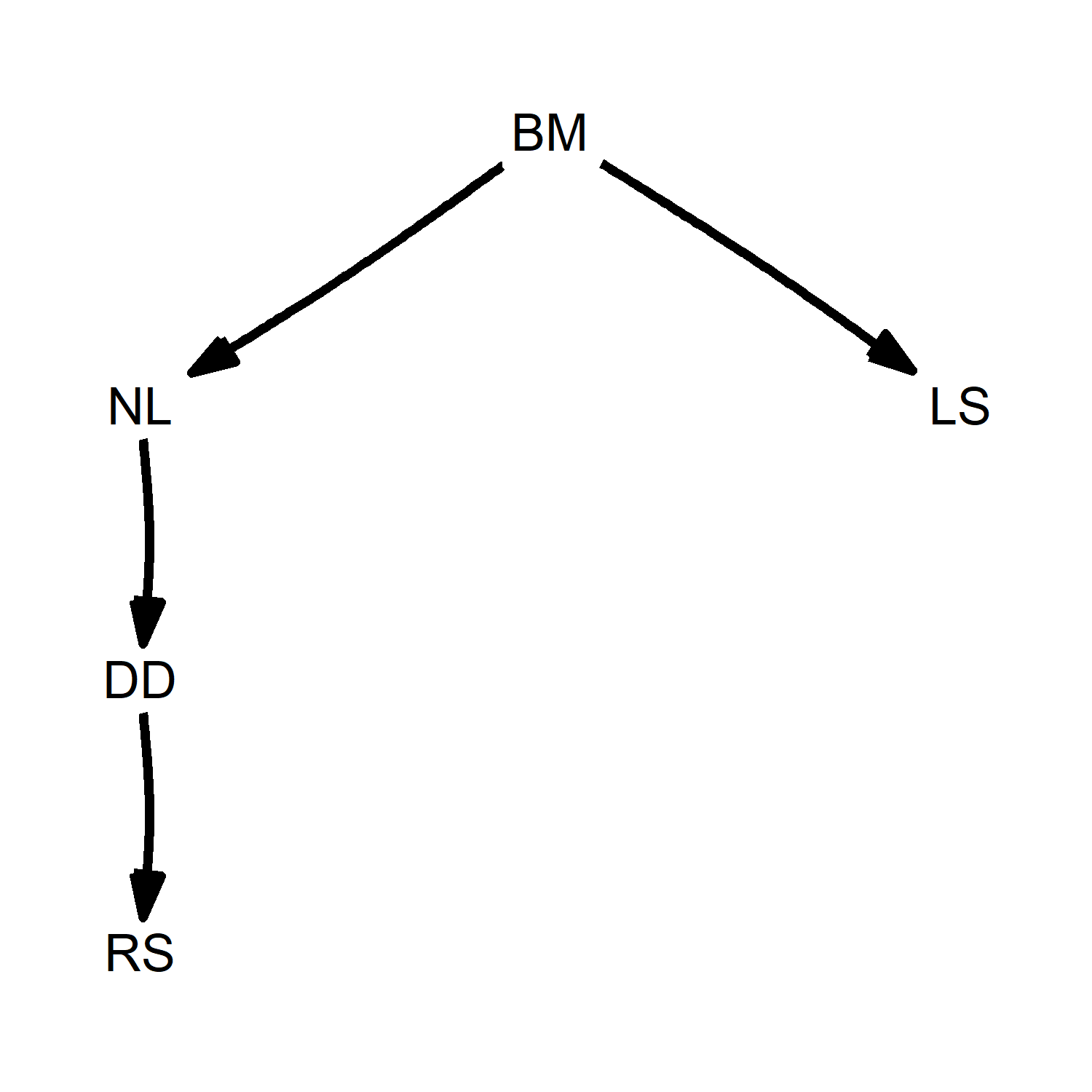Or better yet, plot all of the models at once: plot_model_set(models)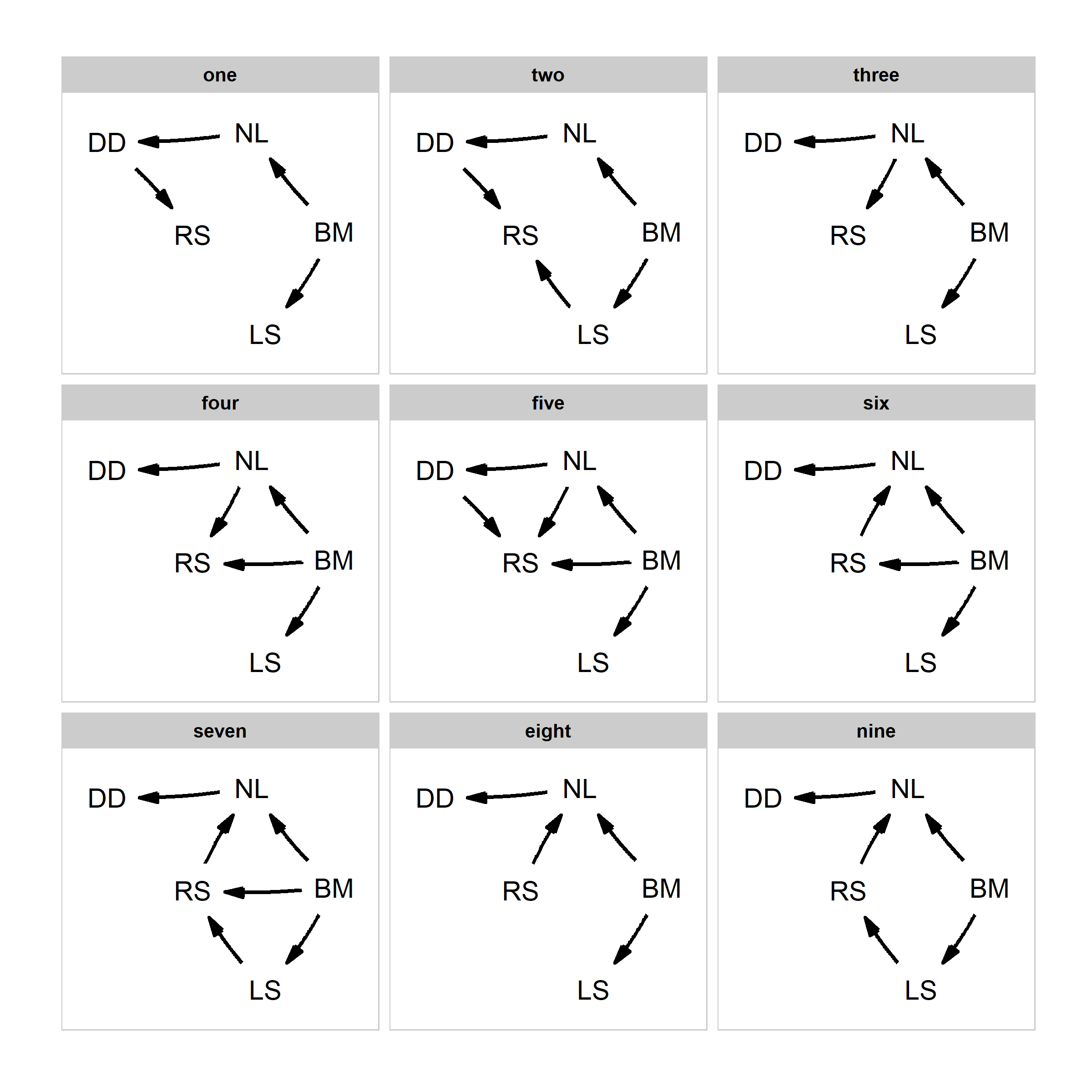### Evaluating the model set Now that we have the model set, we can perform the path analysis using the phylo_path function. For this we will need a data set, included in this package as rhino, as well as a phylogenetic tree, rhino_tree. The package will take care of finding the d-seperation statements and fitting the necessary models. Importantly, when using PGLS, we need to be consistent in which variables are used as independent and dependent variables in the analysis. If one has a specific idea about which variables are to be considered as up- and down-stream, then you can use the order argument to give the ordering (from up to down). In this case, we supply the ordering to mimic the choices made by the chapter authors. Alternatively, you can choose to not supply an order, and the function will try to make a sensible order by itself. If the combination of all causal models is itself a DAG, the ordering of that model will be used, otherwise the ordering will be constructed by consensus (i.e. the most common ordering is chosen). Generally, I advise to not supply the order argument, in order to reduce “researcher degrees of freedom”. By default, phylo_path uses Pagel’s “lambda” model of evolution (specified here for clarity), but if you want, for example, to use a simple Brownian motion model, you can supply model = 'BM' instead. result <- phylo_path(models, data = rhino, tree = rhino_tree, model = 'lambda') The result we end up with is a phylo_path object. Simply printing it gives us a quick summary of what is in the object. In this case we end up with five continuous variables, nine causal models and 21 unique regressions. result ## A phylogenetic path analysis, on the variables: ## Continuous: BM NL DD RS LS ## Binary: ## ## Evaluated for these models: one two three four five six seven eight nine ## ## Containing 46 phylogenetic regressions, of which 21 unique To get an overview of the analysis, we can ask for its summary: (s <- summary(result)) ## model k q C p CICc delta_CICc l w ## 1 eight 6 9 6.113 0.910 26.113 0.000 1.000 0.357 ## 2 six 5 10 4.971 0.893 27.443 1.330 0.514 0.183 ## 3 four 5 10 5.185 0.878 27.657 1.544 0.462 0.165 ## 4 nine 5 10 5.730 0.837 28.202 2.088 0.352 0.125 ## 5 five 4 11 3.456 0.903 28.456 2.342 0.310 0.111 ## 6 seven 4 11 4.702 0.789 29.702 3.589 0.166 0.059 ## 7 three 6 9 27.245 0.007 47.245 21.132 0.000 0.000 ## 8 one 6 9 62.007 0.000 82.007 55.894 0.000 0.000 ## 9 two 5 10 60.967 0.000 83.438 57.325 0.000 0.000 And plotting that summary gives us a quick overview of the support for each model: plot(s)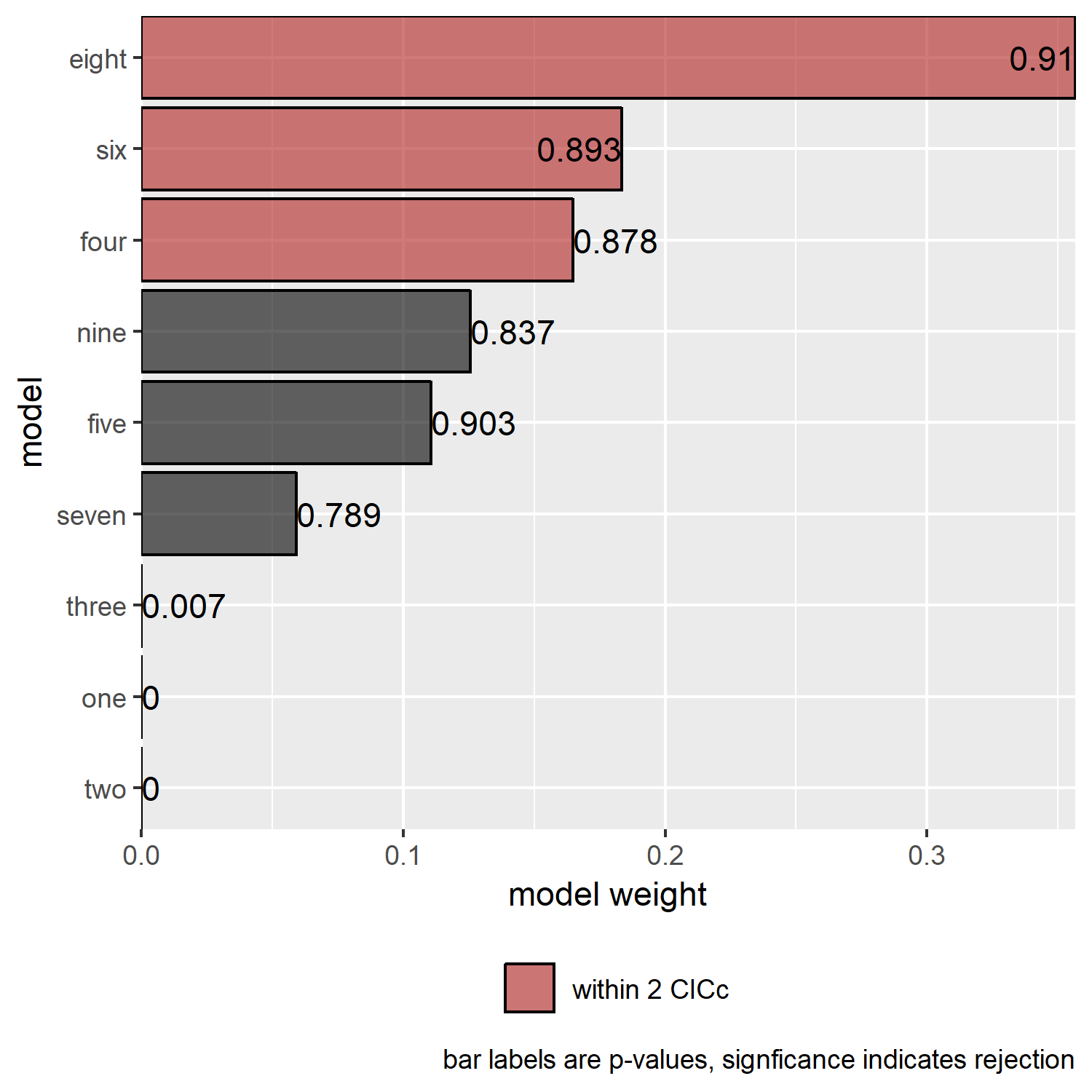### Selecting and fitting a final model To view the best ranked model, we can use best. This returns a DAG with standardized regression coefficients, as well as a matrix of standard errors. These can be obtained for any particular model we looked at by using the choice function. _Note: These functions can also obtain confidence intervals for the estimates, which phylolm achieves through bootstrapping. This makes these very slow though, and by default this is turned off. Pass the number of bootstrap replicates you want to any modelling function in this package via the boot parameter to estimate confidence intervals, e.g. best(result, boot = 500). Either standard errors or confidence intervals can be plotted with coef_plot (see below). (best_model <- best(result)) ##$coef
##    BM        LS RS        NL        DD
## BM  0 0.4973937  0 0.4613623 0.0000000
## LS  0 0.0000000  0 0.0000000 0.0000000
## RS  0 0.0000000  0 0.5280685 0.0000000
## NL  0 0.0000000  0 0.0000000 0.6285344
## DD  0 0.0000000  0 0.0000000 0.0000000
##
## $se ## BM LS RS NL DD ## BM 0 0.08934185 0 0.06500775 0.00000000 ## LS 0 0.00000000 0 0.00000000 0.00000000 ## RS 0 0.00000000 0 0.05726520 0.00000000 ## NL 0 0.00000000 0 0.00000000 0.08006703 ## DD 0 0.00000000 0 0.00000000 0.00000000 ## ## attr(,"class") ##  "fitted_DAG" This object can also be plotted, now the numbers and width of the arrow represent path coefficients. In this case, all paths are green since all relationships are positive. plot(best_model)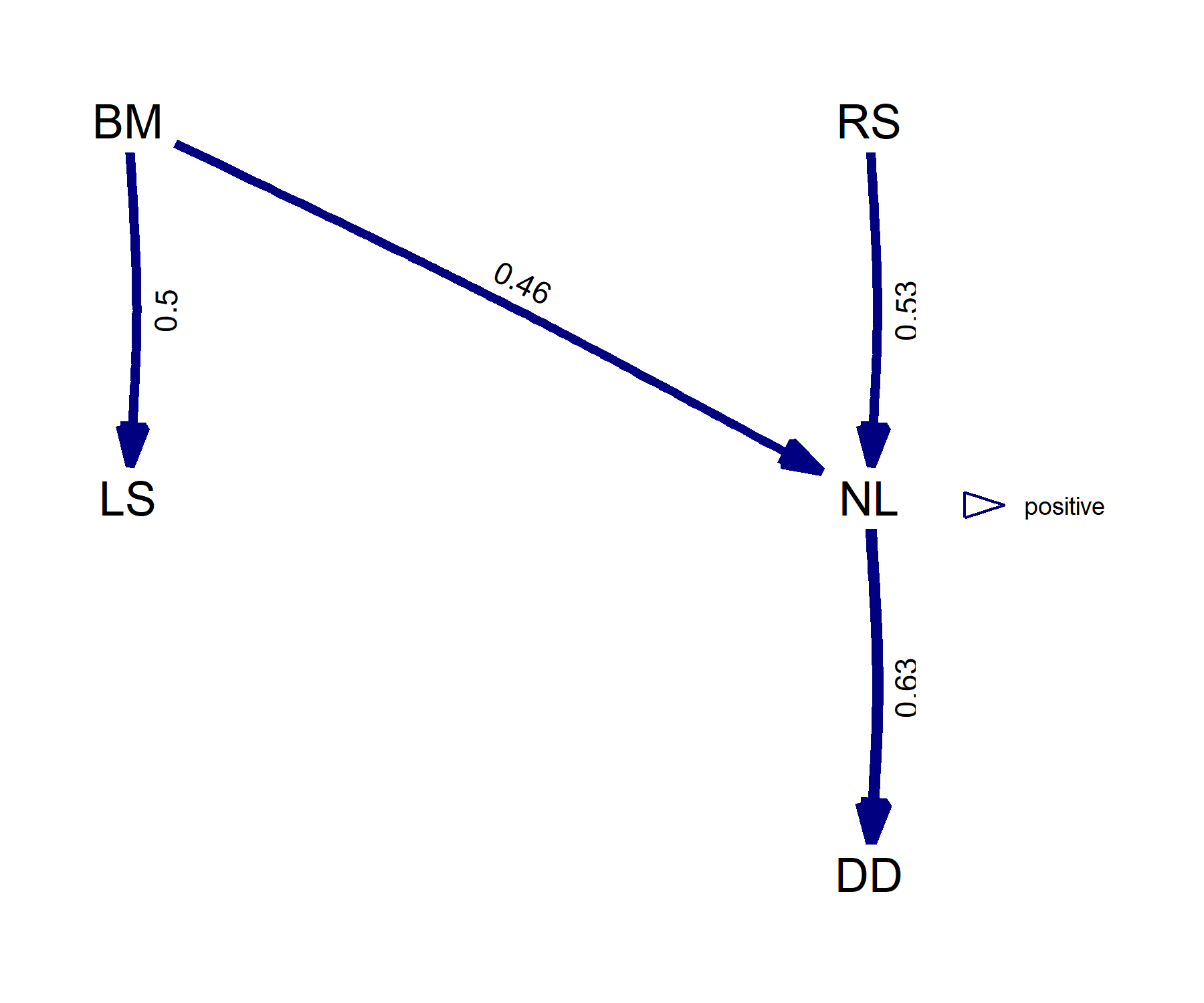From the summary we could see that in reality, there are several models that are quite good. Instead of using the best model, we can use the average of the best models, weighted by their relative evidence. By simply calling average, we can obtain the coefficients and standard errors of the averaged model where the CICc cut_off is 2 by default. If a model does not include a path, we assume that coefficient to be 0. average_model <- average(result) plot(average_model, algorithm = 'mds', curvature = 0.1) # increase the curvature to avoid overlapping edges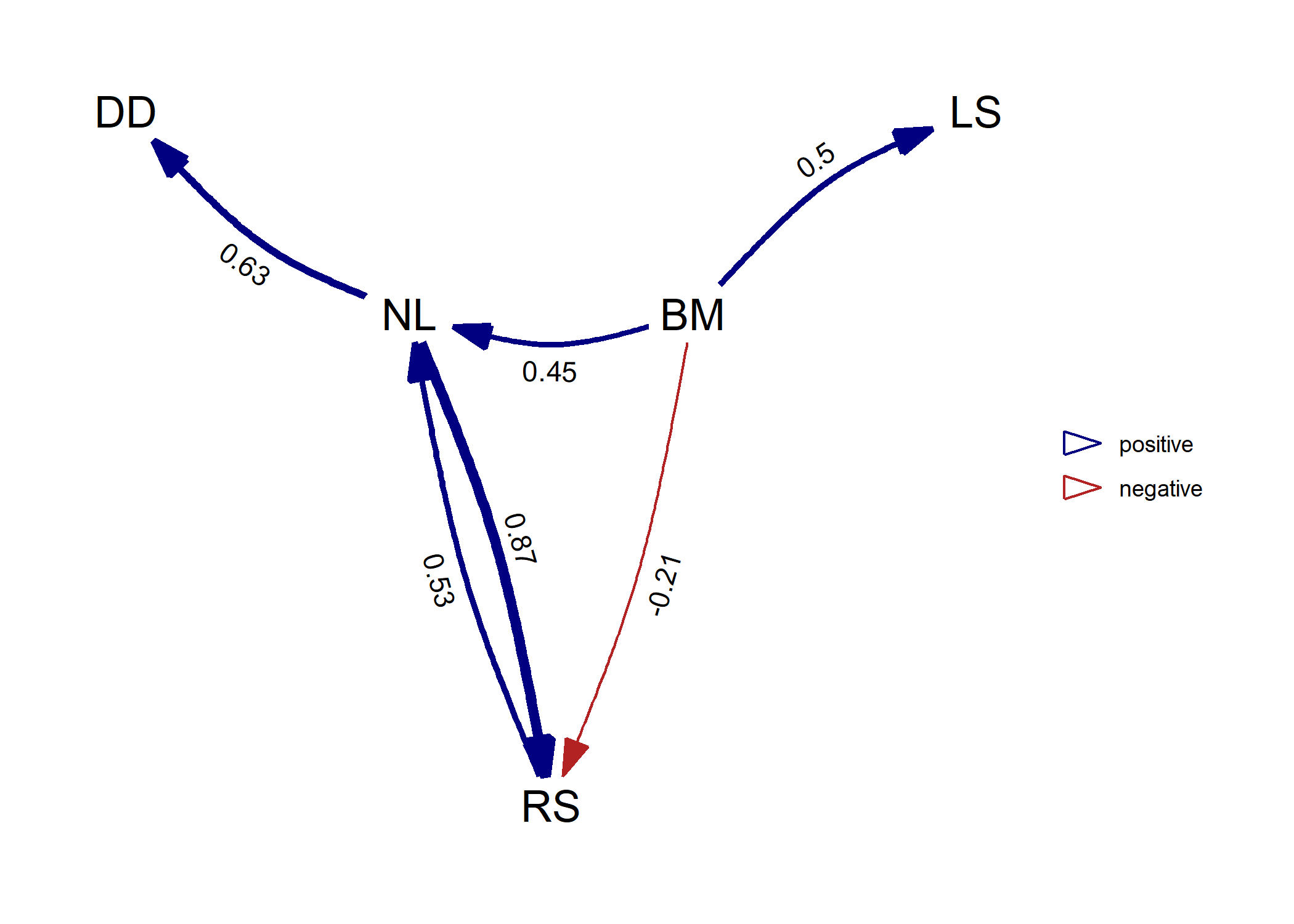Note that, by default, the path averaging is only done for the models that actually contain that path. This facilitates the detection of weak effects, but also biases coefficients away from zero. Alternatively, we can assume the coefficients (and their variance) for absent paths to be zero by setting avg_method = "full". average_model_full <- average(result, avg_method = "full") plot(average_model_full, algorithm = 'mds', curvature = 0.1)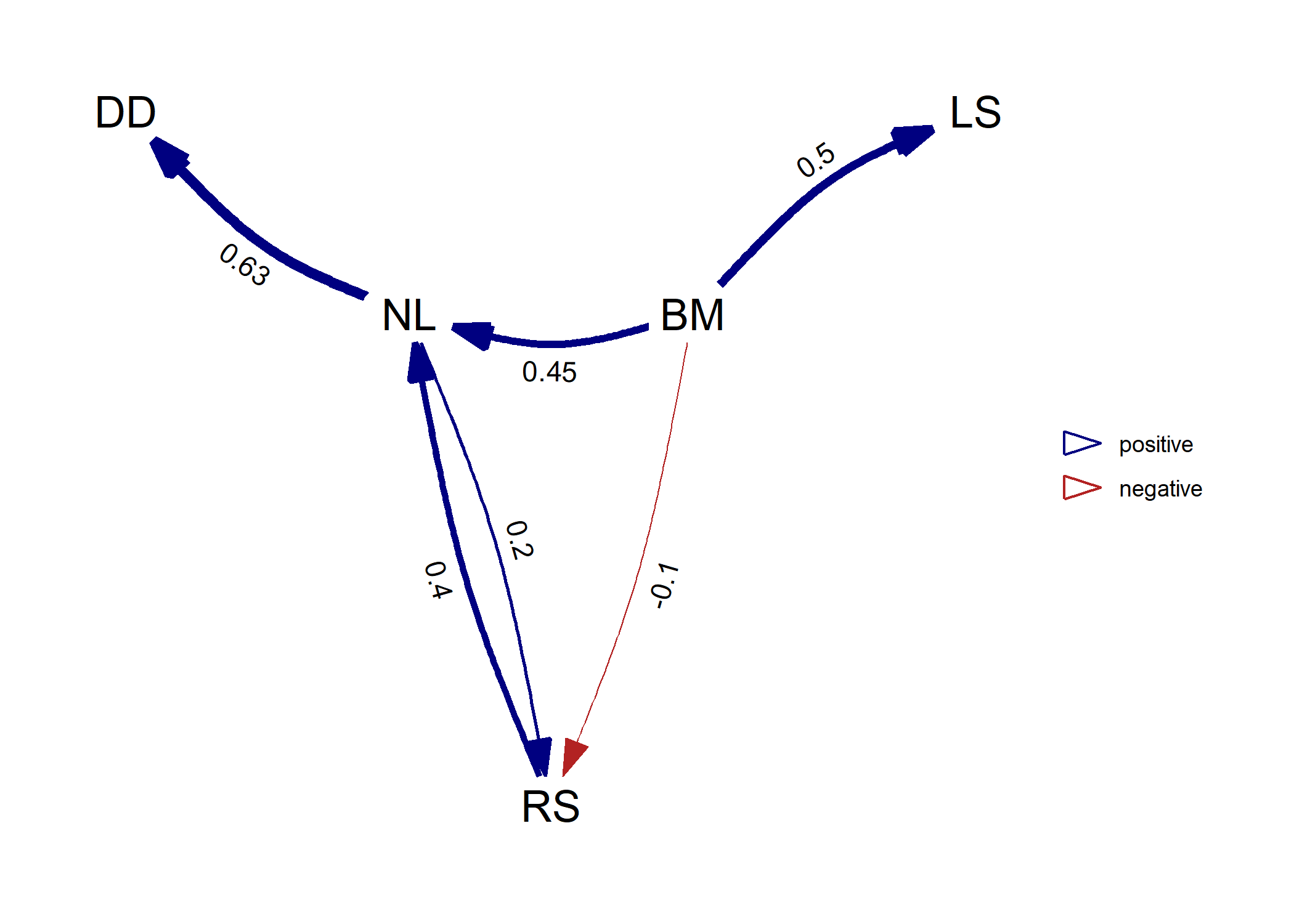We can see that paths that occur in all the best models, such as NL -> DD, are not effected. But paths that occur only in some models, such as NL -> RS, suffer shrinkage reflecting the fact that they were not as well supported. In this case in particular, the average model is actually not a DAG since it is cyclical and the relationship between RS and NL is clearly not well resolved by our analysis. If one is interested in the confidence in the regression coefficients, coef_plot can visualize the estimates and their approximate confidence intervals (you need to use the boot parameter when fitting the DAG if you are not model averaging, see above). We can see that for the . The order of the paths from left to right, attempts to follow the paths downstream, in this indicated by the order argument we gave the phylo_path function at the start. #coef_plot(average_model) This plot, and others in the package, can be manipulated using ggplot2 functions, for example, to create a horizontal black-and-white version. We can see that for full average model, shrinkage has caused several paths to become uncertain. # coef_plot(average_model_full, reverse_order = TRUE) + # ggplot2::coord_flip() + # ggplot2::theme_bw() Finally, you can access the conditional independencies and their associated p-values as well. This can be useful if you want to know why a certain model was rejected. The phylo column gives us the estimates for the correlation structure for each of the fitted models, in this case lambda since we used corPagel. For binary models this shows s2, which also reflects the strength of phylogenetic signal. Finally, all models are stored in the model list as well, in case other information needs to be extracted. result$d_sep\$one
## # A tibble: 6 x 4
##   d_sep                    p phylo_par model
##   <chr>                <dbl>     <dbl> <list>
## 1 DD ~ NL + BM      4.69e- 1     0.374 <S3: phylolm>
## 2 RS ~ DD + BM      2.46e- 1     0.529 <S3: phylolm>
## 3 RS ~ BM + DD + NL 5.40e-13     0.620 <S3: phylolm>
## 4 NL ~ BM + LS      9.94e- 1     0.778 <S3: phylolm>
## 5 DD ~ NL + BM + LS 5.69e- 1     0.403 <S3: phylolm>
## 6 RS ~ DD + BM + LS 9.74e- 1     0.528 <S3: phylolm>

For model 1 it seems that the third conditional independence statement was violated (it has a very small p-value).